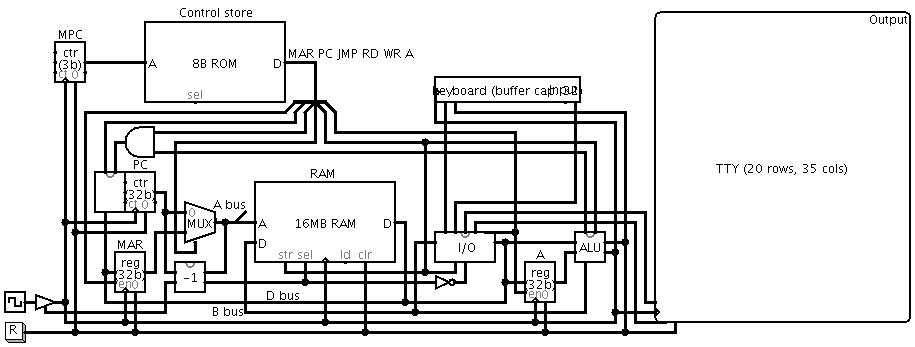# davidar/subleq

No description, website, or topics provided.Fetching latest commit…
Cannot retrieve the latest commit at this time.
Type Name Latest commit message Commit time
Failed to load latest commit information.jsubleqlogisimsrcthirdpartyutilCOPYINGMakefileREADMEREADME.md

SUBLEQ (SUbtract and Branch if Less than or EQual to zero) is a type of OISC (One Instruction Set Computer) / URISC (Ultimate Reduced Instruction Set Computer) architecture. It has only one instruction of the form:

``````A B C
``````

This is equivalent to the following pseudocode:

```mem[B] = mem[B] - mem[A]
if mem[B] <= 0 then goto C```

Compound instructions, such as addition, can be formed from sequences of these simple SUBLEQ instructions. For examples of these, see the source files - most compound instructions include a comment about their function. Indirect addressing (pointers) can be emulated through self-modifying code, where operations are performed on the instructions themselves. See (1,2,3,4,6) for further information.

This project consists of four main parts:

• a CPU capable of executing SUBLEQ instructions
• libraries for programs written in SUBLEQ assembly, and a demo program to demonstrate these
• a minimal SUBLEQ interpreter (with I/O) written in C
• JSubleq: a SUBLEQ interpreter written in JavaScript

### CPU

Schematics for a SUBLEQ-based CPU are provided.A recent version of Logisim is required to simulate the CPU. Simulation instructions are provided below.

There are three main buses in the CPU:

• A bus: contains the address of the current memory location
• B bus: contains the output of the ALU
• D bus: contains the data read from memory

The CPU has six control signals:

• MAR: the Memory Address Register should be updated with the value of the D bus at the end of this cycle
• PC: the Program Counter should be updated at the end of this cycle
• JMP: the PC should be loaded with the value of the D bus at the end of this cycle if the LEQ flag has been set by the ALU, otherwise PC is just incremented if either JMP or LEQ are false
• RD: the value of MAR should be loaded onto the A bus, otherwise it is loaded with the value of PC if RD is false
• WR: the value of the B bus should be stored to memory at the end of this cycle, and the value of the LEQ flag should be updated
• A: signals that the "A" operand is currently on the D bus, so the A register should be updated at the end of this cycle

These signals are controlled by a 5-microinstruction microprogram which runs in a continous loop. The signals enabled by each of these microinstructions are:

1. MAR, PC: load `mem[PC]` (address of A operand) into MAR, increment PC
2. RD, A: load `mem[MAR]` into the A register
3. MAR, PC: load `mem[PC]` (address of B operand) into MAR, increment PC
4. RD, WR: load `mem[MAR]` onto the D bus, then write the value of the B bus (equal to the value of the D bus minus the value of the A register) into `mem[MAR]`
5. PC, JMP: if the LEQ flag is set (the result of B-A was less than or equal to zero) then set PC to `mem[PC]` (the C operand) i.e. jump to C, otherwise increment PC

Input/output is performed through memory-mapped I/O, with the I/O driver mapped to address 0xffffffff (-1). If the A signal is enabled, then reading from this address returns the negation of the next input character. If the A signal is not enabled, then the value 0xffffffff is returned. Writing to this address prints the character obtained by performing a bitwise NOT on the value of the B bus. This behaviour allows the following I/O instructions to be performed:

• (-1) X: add the input character to X (X -= -input)
• X (-1): output the character in X (subtracting X from 0xffffffff gives NOT X, then performing a NOT on this yields the original X)

Jumping to address 0xffffffff (-1) disables the clock signal, effectively halting the CPU.

#### Simulation

To simulate the CPU:

1. open cpu.circ in Logisim
2. increase the simulation speed: Simulate > Tick Frequency > 1024 Hz
3. load a program: to run the demo program, first make sure you have run `make` in the project root directory, then right-click the component labeled "RAM" > Load Image... > ../image.hex
4. start the simulation: Simulate > Ticks Enabled
5. make sure you're using the hand tool, and select the component labeled "Input" by clicking on it
6. interact with the program as you would with any other console-based application
7. if the clock line turns blue, it means that the program has halted
8. to stop the machine, uncheck Simulate > Ticks Enabled, then press the reset button labeled "R"
9. repeat from step 3 if necessary

### Libraries/Demo

Libraries for programs written in SUBLEQ assembly, as accepted by sqasm, are available.

They provide several common functions:

• getint (read integer from input)
• gets (read string from input)
• putint (write integer to output)
• puts (write string to output)

Several useful procedures are also available:

• bubblesort (sort a string of characters)
• calc (perform a given operation on two integers)
• factorial (calculate the factorial of a positive integer)
• primes (generate and print a list of primes)

Usage information and equivalent C code (where appropriate) is provided in the comments of each of these files.

A menu-driven program demonstrating the above libraries is also provided. Simply call `make run` in the project root directory to run the demo program.

### Interpreter

A very minimal (only 222 bytes in size) SUBLEQ interpreter written in C is available:

```#include<stdio.h>
main(int C,char**A){FILE*F=fopen(A,"r");int P=0,_,*i=_;while(fscanf(F,
"%d",i++)>0);while(P>=0){int a=_[P++],b=_[P++],c=_[P++];a<0?_[b]+=getchar():b<0
?printf("%c",_[a]):(_[b]-=_[a])<=0?P=c:0;}}```

It has been somewhat obfuscated to reduce the code size, so a more readable version is also provided. It should function similarly to sqrun.

### JSubleq

This terminal demonstrates the JavaScript interpreter running the provided demo program.

You can’t perform that action at this time.